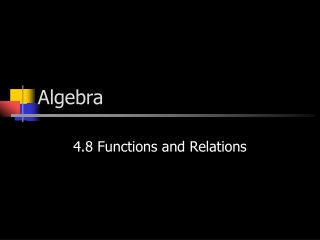DownloadDownload PresentationAlgebra

# Algebra

Télécharger la présentation## Algebra

- - - - - - - - - - - - - - - - - - - - - - - - - - - E N D - - - - - - - - - - - - - - - - - - - - - - - - - - -
##### Presentation Transcript

1. Algebra 4.8 Functions and Relations

2. Functions and Relations • A relation is a set of ordered pairs • A function is a rule that establishes a relationship between two quantities, called the input and the output. • Input = x The collection of the x values is known as the domain. • Output = y. The collection of the y values is known as the range.

3. Function • The most important part of the function: every input has only one output. An x cannot have two y’s. • Examples: y y x x 1 5 1 2 1 7 2 4 2 8 3 4 3 9 4 5 Is this relation a function? Is this relation a function? No. The input 1 has Two outputs 5 and 7. Yes. Each input has only one output.

4. A Birthday Example • Imagine this relation: • Input = All the students at CHSFA • Output = The Birthday of that Student • Is this a function?

5. Let’s See Birthday Person Is this a function? Jessica Jones May 5th Does each input have only one output? Bob Boone June 21st Similarly, does each person have only one birthday? Sally Smith Nov. 18th Todd Thomas Nov. 18th Since each input has only one output, then this is a function. Todd Thomas April 3rd This would make this not a function because an input (Todd Thomas) would have more than one Output (Nov. 18th and April 3rd). This cannot happen.

6. What if the input were the days of the year and the output was the people who had that day as their birthday?Let’s try as a class. People’s B-Day Date Is this a function? Jan. 1st Stu Sanders Does each input have only one output? Jan. 2nd Lyn Lewis Similarly, does each date have only one person celebrating that day as their birthday? Jan. 3rd John Jacobs Jan. 3rd Sally Struthers No. Since there is an input that has more than one output, then this is not a function.

7. Vertical Line Test A relation is a function if its graph passes the vertical line test. All vertical lines must intersect the graph only once. Are these functions? Do they pass the vertical line test? Yes! No! No! Yes!

8. Evaluate the Following Function in Function Notation • f(x) = 2x – 3 when x = -2 f(-2) = 2(-2) – 3 = -4 – 3 = -7

9. You try! Evaluate the Following Function in Function Notation… • f(x) = -7x – 3 when x = 4 f(4) = -7(4) – 3 = -28 – 3 = -31

10. Graphing a Function • Graph f(x) = -1/2x + 4 • Replace f(x) with y • Graph y = -1/2x + 4 (0, 4)

11. Is the relation a function? If yes, state the domain and range. y x Yes, the relation is a function! 1 -2 2 -3 The domain is 1, 2, 3, and 4 3 -3 4 -5 The range is -2, -3, -3, -5

12. Real-world functions • Coke machine • Toaster • Lets come up with a few as a class.

13. Function • Function- exactly one output for one input.

14. Function Rule • Function Rule- equation that describes a functional relationship. • X= total cokes • 1= price per coke • N= number of cokes needed x=1(n)

15. Writing a function rule Input (x) output (y)

16. Find the difference between each box 1 1 1 X= Y= 2.75 2.75 2.75

17. Put into fraction form and divide Y 2.75 2.75 = = 1 X

18. Plug into equation y=#(x) y=2.75x

19. Check it... y=2.75x 2.75 (3) y= 8.25= 2.75 (3)

20. What if it doesn’t work? 6 6 = 1 6 1 1 6 1 6

21. What if it doesn’t work??? y=6(x) y=6(2) 12= 6(2) ?

22. What do I need to add? y=6(2)+___ 16=6(2)+___ 4 16= 12+4

23. The Rule is. Check it with another number. y=6x+4 28=6(4)+4 ? YES!!!